NEET  >  Doppler's Effect

# Doppler's Effect | Physics Class 11 - NEET

 1 Crore+ students have signed up on EduRev. Have you?

11. Doppler's Effect

When a car at rest on a road sounds its high frequency horn and you are also standing on the road near by, you'll hear the sound of same frequency it is sounding but when the car approaches you with its horn sounding, the pitch (frequency) of its sound seems to drop as the car passes. This phenomenon was first described by an Australian Scientist Christian Doppler, is called the Doppler effect, He explained that when a source of sound and a listener are in motion relative to each other, the frequency of the sound heard by the listener is not the same as the source frequency. Lets discuss the Doppler effect in detail for different cases.

11.1 Stationary Source and Stationary Observer

Figure shown a stationary sources of frequency n0 which produces sound waves in air of wavelength l0 given as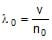[v = speed of sound in air]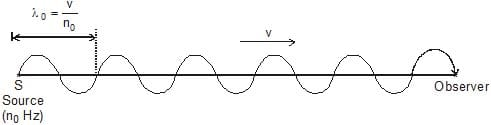Although sound waves are longitudinal, here we represent sound weaves by the transverse displacement curve as shown in figure to understand the concept in a better way. As source produces waves, these waves travel towards, stationary observer O in the medium (air) with speed v and wavelength l0. As observer is at rest here it will observe the same wavelength l0 is approaching it with speed v so it will listen the frequency n given as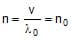[same as that of source] ...(1)

This is why when a stationary observer list ends the sound from a stationary source of sound, it detects the same frequency sound which the source is producting. Thus no Doppler effect takes place if there is no relative motion between source and observer.

11.2 Stationary Source and Moving Observer

Figure shown the case when a stationary sources of frequency n0 produces sound waves which have wavelength in air given as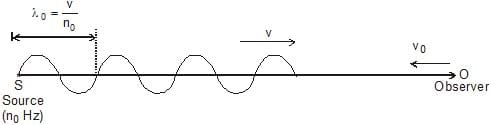These waves travel toward moving observer with velocity v0 towards, the source. When sound waves approach observer, it will receive the waves of wavelength l0 with speed v v0 (relative speed). Thus the frequency of sound heard by observer can be given as

Apparent frequency nap =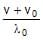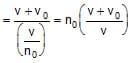...(2)

Similarly we can say that if the observer is receding away from the source the apparent frequency heard by the observer will be given as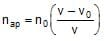...(3)

11.3 Moving Source and Stationary Observer

Figure shows the situation when a moving source S of frequency n0 produces sound waves in medium (air) and the waves travel toward observer with velocity v.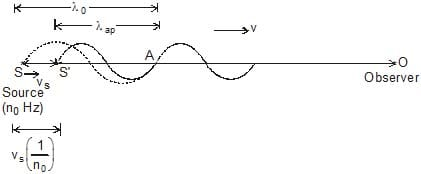Here if we carefully look at the initial situation when source starts moving with velocity vs as well as it starts producting waves. The period of one oscillation is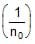sec and in this duration source emits one wavelength l0 in the direction of propagation of waves with speed v, but in this duration the source will also move forward by a distance vs. Thus the effective wavelength of emitted sound in air is slightly compressed by this distance as shown in figure. This is termed as apparent wavelength of sound in medium (air) by the moving source. This is given as

Apparent wavelength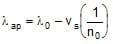...(1)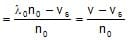Now this wavelength will approach observer with speed v ( O is at rest). Thus the frequency of sound heard by observer can be given as

Apparent frequency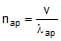=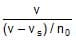=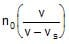...(2)

Similarly if source is receding away from observer, the apparent wavelength emitted by source in air toward observer will be slightly expanded and the apparent frequency heard by the stationary observer can be given as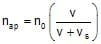...(3)

11.4 Moving Source and Moving Observer

Let us consider the situation when both source and observer are moving in same direction as shown in figure at speeds vs and v0 respectively.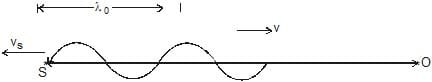In this case the apparent wavelength emitted by the source behind it is given as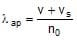Now this wavelength will approach the observer at relative speed v v0 thus the apparent frequency of sound heard by the observer is given as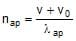=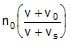...(1)

By looking at the expression of apparent frequency given by equation, we can easily develop a general relation for finding the apparent frequency heard by a moving observer due to a moving source as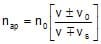...(2)

Here + and - signs are chosen according to the direction of motion of source and observer. The sign convention related to the motion direction can be stated as :

(i) For both source and observer v0 and vs are taken in equation with -ve sign if they are moving in the direction of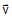i.e. the direction of propagation of sound from source to observer.

(ii) For both source and observer v0 and vs are taken in equation (2) with ve sign if they are moving in the direction opposite toi.e. opposite to the direction of propagation of sound from source to observer.

11.5 Doppler Effect in Reflected Sound

When a car is moving toward a stationary wall as shown in figure. If the car sounds a horn, wave travels towards the wall and is reflected from the wall. When the reflected wave is heard by the driver, it appears to be of relatively high pitch. If we wish to measure the frequency of reflected sound then the problem must be handled in two steps.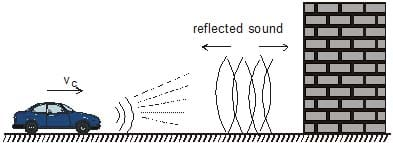First we treat the stationary wall as stationary observer and car as a moving source of sound of frequency n0. In this case the frequency received by the wall is given as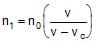...(1)

Now wall reflects this frequency and behaves like a stationary source of sound of frequency n1 and car (driver) behave like a moving observer with velocity vc. Here the apparent frequency heard by the car driver can be given as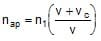=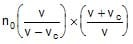=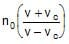(2)

Same problem can also be solved in a different manner by using method of sound images. In this procedure we assume the image of the sound source behind the reflector. In previous example we can explain this by situation shown in figure.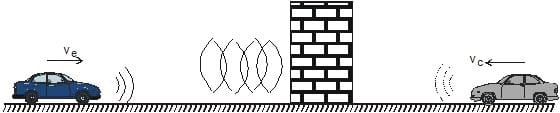Here we assume that the sound which is reflected by the stationary wall is coming from the image of car which is at the back of it and coming toward it with velocity vc. Now the frequency of sound heard by car driver can directly be given as

nap = n0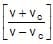...(3)

This method of images for solving problems of Doppler effect is very convenient but is used only for velocities of source and observer which are very small compared to the speed of sound and it should not be used frequently when the reflector of sound is moving.

11.6 Doppler's Effect for Accelerated Motion

For the case of a moving source and a moving observer, we known the apparent frequency observer can be given as...(4)

Here v is the velocity of sound and v0 and vs are the velocity of observer and source respectively.

When a source of observer has accelerated or retarded motion then in equation (4) we use that value of v0 at which observer receives the sound and for source, we use that value of vs at which it has emitted the wave.

The alternative method of solving this case is by the traditional method of compressing or expending wavelength of sound by motion of source and using relative velocity of sound with respect to observer

11.7 Doppler's Effect when Source and Observer are not in Same Line of Motion

Consider the situation shown in figure. Two cars 1 and 2 are moving along perpendicular roads at speed v1 and v2. When car - 1 sound a horn of frequency n0, it emits sound in all directions and say car - 2 is at the position, shown in figure. when it receives the sound. In such cases we use velocity components of the cars along the line joining the source and observer thus the apparent frequency of sound heard by car-2 can be given as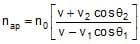...(6.266)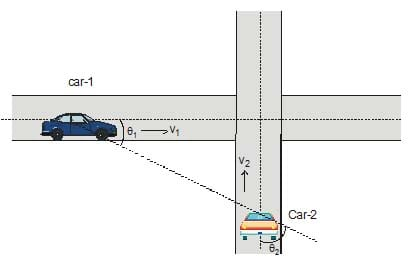The document Doppler's Effect | Physics Class 11 - NEET is a part of the NEET Course Physics Class 11.
All you need of NEET at this link: NEET

## Physics Class 11

124 videos|464 docs|210 tests

## Physics Class 11

124 videos|464 docs|210 tests

### How to Prepare for NEET

Read our guide to prepare for NEET which is created by Toppers & the best Teachers

Track your progress, build streaks, highlight & save important lessons and more!(Scan QR code)

,

,

,

,

,

,

,

,

,

,

,

,

,

,

,

,

,

,

,

,

,

;